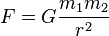# Electric/Gravitational Forces

thst1003
Find the ratio of the magnitudes of the electrical and the gravitational forces acting between a proton and an electron separated by an arbitrary distance d.

F= k(q)(q')/r^2 andThe q's are in an absolute value sign. How do I find the ratio? Is it as simply as dividing it out?

Last edited:

Staff Emeritus
Gold Member
The q's are in an absolute value sign. I believe there is an additional equation similar to this one that deals with gravity that I need to use as well but I can't find it.

Although I have a lot of trouble believing that you have not encountered this already, I will just take your post at face value. Here is the equation you seek:

http://en.wikipedia.org/wiki/Newton's_law_of_universal_gravitation

thst1003
I changed the question. I have encountered it.

Aquinox
that's a trivial problem asked on electrostatics. Yep, you divide it for a ratio.

thst1003
What exactly do you divide? Is it Electrical force/Gravitational force using 1.6e-19 C for the electron and the electron? So it would be k(q)(q) / G(m1)(m2) ???

Staff Emeritus